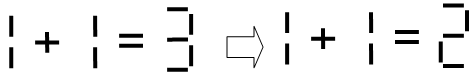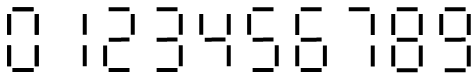## Day 1, Problem 1 - Stick Game

This is a very ancient game. The objective is to arrange sticks on a table to create an equation that does not hold true, in a way so that if a single stick is moved, the equation will become true. Now it is your turn.Figure 1. moving one stick to make an equation valid

You are given one equation that is guaranteed to not hold true. If it is possible to to make the equation true by moving a single stick, then output the new equation. Otherwise, output "`No`" (without quotes).

The following rules and constraints hold for the equation:

1. The equation may contain positive or negative numbers. The only operators will be the plus sign and the minus sign. There will only be one equal sign.
2. The equation will not contain numbers with 10 or more digits (all operands will be less than or equal to 999999999 in absolute value).
3. You may only move sticks used to construct numbers, not sticks used for the operators (+, −, =). Therefore the plus, minus, and equal signs will not change. Before and after moving a stick, the arrangements of each digit must strictly correspond to that of figure 2.
4. There will not be leading zeros for numbers in the initial equation, but leading zeros in numbers after moving a stick is permitted.Figure 2. ways to express the digits 0-9 using sticks

### Input Format

The input will contain one equation on a single line, followed by the single character '`#`' (ASCII code 35). There will not be spaces or other separator characters within the equation. The length of equation will be less than or equal to 1000 characters. Note: After the '`#`' character, there may be characters that are irrelevant to the problem.

### Output Format

The output should contain one line, the corrected equation, in the same format as the input (terminated with a '`#`', with no extra characters anywhere in the output). If a solution does not exist, output "`N`o" (uppercase `N`, lowercase `o`, without quotes).

`1+1=3#`

`1+1=2#`

### Sample Input 2

`1+1=3+5#`

`No`

### Sample Input 3

`11+77=34#`

### Sample Output 3

`17+17=34#`

Point Value: 15 (partial)
Time Limit: 1.00s
Memory Limit: 64M